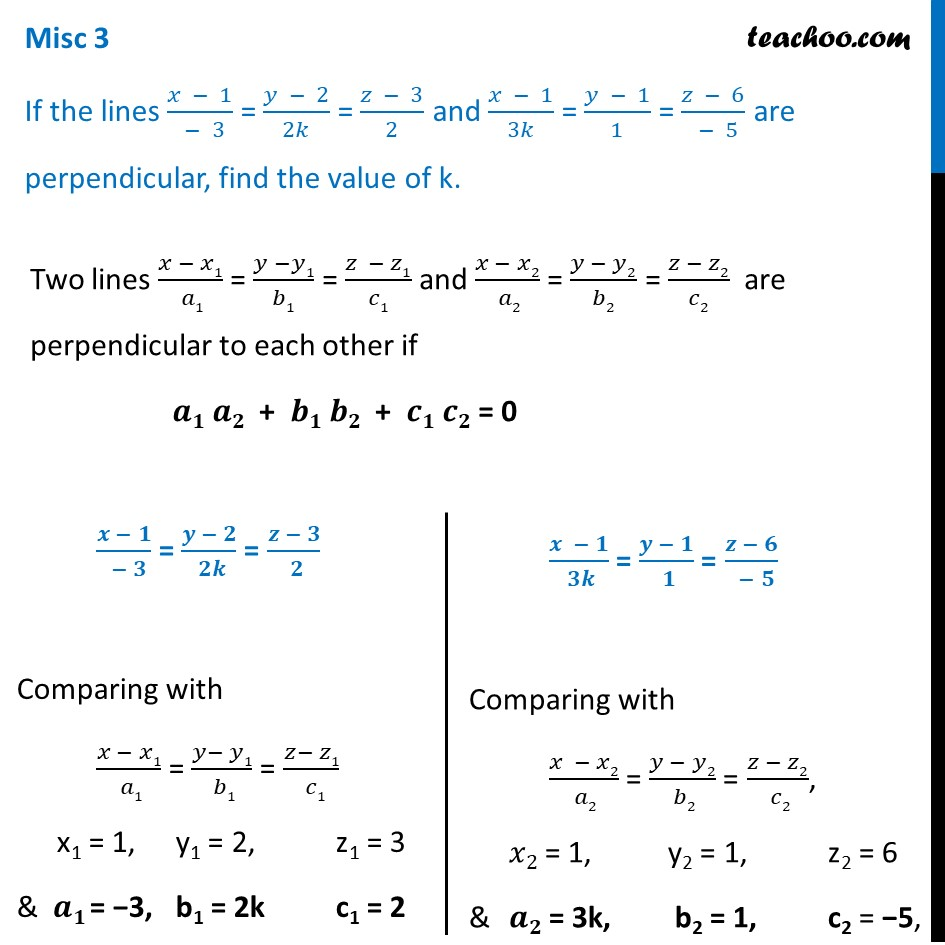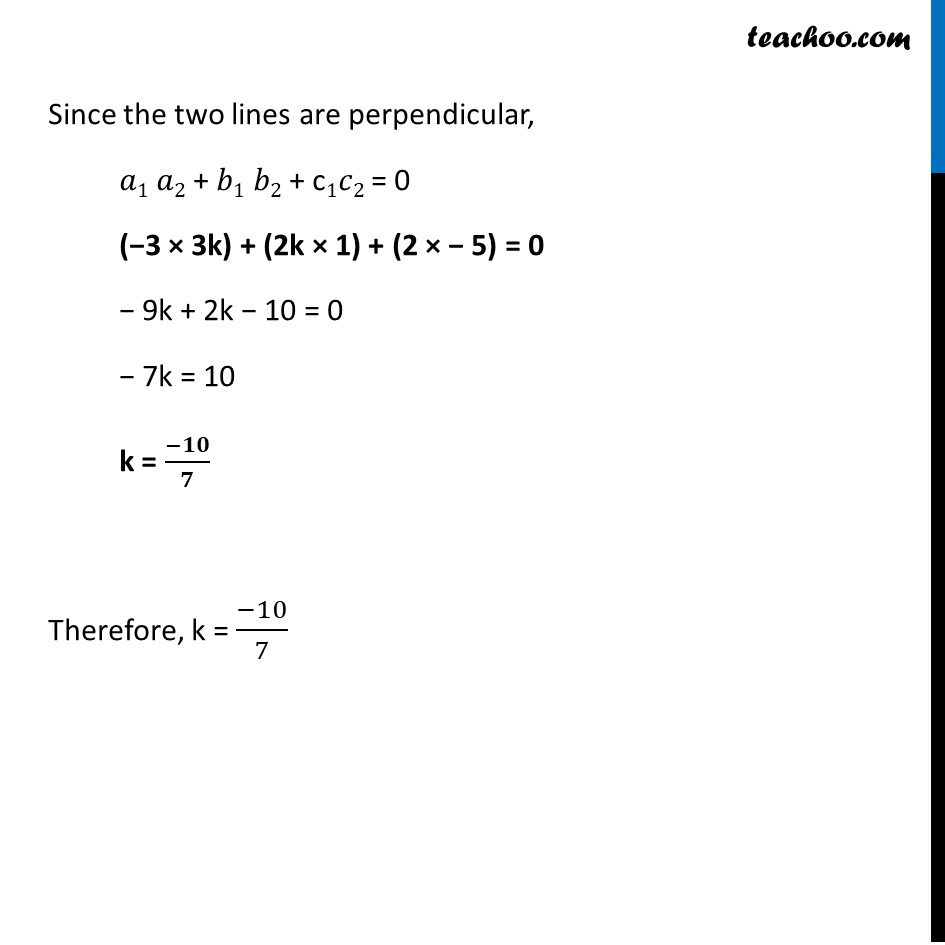Miscellaneous

Chapter 11 Class 12 Three Dimensional Geometry
Serial order wiseLearn in your speed, with individual attention - Teachoo Maths 1-on-1 Class

### Transcript

Misc 3 If the lines (𝑥 − 1)/( − 3) = (𝑦 − 2)/2𝑘 = (𝑧 − 3)/2 and (𝑥 − 1)/3𝑘 = (𝑦 − 1)/1 = (𝑧 − 6)/( − 5) are perpendicular, find the value of k. Two lines (𝑥 − 𝑥1)/( 𝑎1) = (𝑦 −𝑦1)/𝑏1 = (𝑧 − 𝑧1)/𝑐1 and (𝑥 − 𝑥2)/( 𝑎2) = (𝑦 − 𝑦2)/𝑏2 = (𝑧 − 𝑧2)/𝑐2 are perpendicular to each other if 𝒂𝟏 𝒂𝟐 + 𝒃𝟏 𝒃𝟐 + 𝒄𝟏 𝒄𝟐 = 0 (𝒙 − 𝟏)/( − 𝟑) = (𝒚 − 𝟐)/( 𝟐𝒌) = (𝒛 − 𝟑)/( 𝟐) Comparing with (𝑥 − 𝑥1)/( 𝑎1) = (𝑦− 𝑦1)/( 𝑏1) = (𝑧− 𝑧1)/( 𝑐1) x1 = 1, y1 = 2, z1 = 3 & 𝒂𝟏 = −3, b1 = 2k c1 = 2 (𝒙 − 𝟏)/( 𝟑𝒌) = (𝒚 − 𝟏)/( 𝟏) = (𝒛 − 𝟔)/( − 𝟓) Comparing with (𝑥 − 𝑥2)/( 𝑎2) = (𝑦 − 𝑦2)/( 𝑏2) = (𝑧 − 𝑧2)/( 𝑐2), 𝑥2 = 1, y2 = 1, z2 = 6 & 𝒂𝟐 = 3k, b2 = 1, c2 = −5, Since the two lines are perpendicular, 𝑎1 𝑎2 + 𝑏1 𝑏2 + c1𝑐2 = 0 (−3 × 3k) + (2k × 1) + (2 × − 5) = 0 − 9k + 2k − 10 = 0 − 7k = 10 k = (−𝟏𝟎)/𝟕 Therefore, k = (−10)/7